Maths-
General
Easy

Question

# If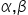be that roots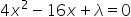where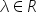,  such that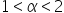and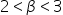then the number of integral solutions of λ is

## 562    3Hint:

## The correct answer is: 3

### A quadratic equation, or sometimes just quadratics, is a polynomial equation with a maximum degree of two. It takes the following form:ax² + bx + c = 0where a, b, and c are constant terms and x is the unknown variable.Now we have givenare the roots of equationwhere,  such thatand.The equation is:D=b2-4ac Applying it, we get:D=162−16λ>0λ<16...........................(i)Now, we have sum of roots as: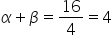we have product of the roots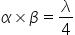Now as per the condition, we have:and, multiplying both, we get:Here we used the concept of quadratic equations and solved the problem. We also understood the concept of discriminant and used it in the solution to find the intervals. Therefore, the number of integral solutions of λ is in between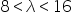#### With Turito Foundation.#### Get an Expert Advice From Turito.